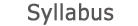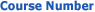0351-2202 Thermodynamics2019 - 2020View groups
Course description

• The context of thermodynamics (large systems)
• Mathematical reminder: derivative, partial derivative, differential, exact differential
• Concepts and definitions: state function, thermodynamic variables, intensive and extensive quantities, systems in contact, thermodynamic equilibrium, thermodynamic process, thermodynamic cycle, Zeroth Law of thermodynamics
• Equations of state of gases: ideal gas, virial expansion, van der Waals gas
• First Law of thermodynamics: work, heat, heat capacity, enthalpy
• Thermodynamic processes in ideal gases: isothermal expansion, isobaric expansion, isochoric heating, adiabatic expansion, free irreversible expansion
• Thermochemistry: heat of reaction, Hess law
• Second Law of thermodynamics: entropy, spontaneous process, entropy change in reversible and irreversible processes, entropy of mixing, statistical interpretation
• Heat engines, heat pumps
• Third Law of thermodynamics
• Thermodynamic potentials: Legendre transform, Helmoholtz free energy, Gibbs free energy, grand-canonical potential, fundamental equations, natural variables, criteria for spontaneous processes, Maxwell relations, fugacity, activity
• Chemical equilibrium: degree of reaction, reactive free energy, equilibrium constant, van't Hoff equation, reactions in gas phase
• Phase transitions: gas-liquid transition, critical point, law of corresponding states, phase coexistence, equal-area construction, lever rule, latent heat, metastable phases, phase diagrams, Clapeyron and Clausius-Clapeyron equations, Gibbs phase rule
• Binary mixtures: bubble and dew lines, Raoult's law, Henry's law, activity coefficients, osmotic pressure
• Electrochemistry: activity of electrolytes, Debye-Hückel theory, electrochemical equilibrium, electrochemical cell, applications to thermodynamic measurements

accessibility declaration